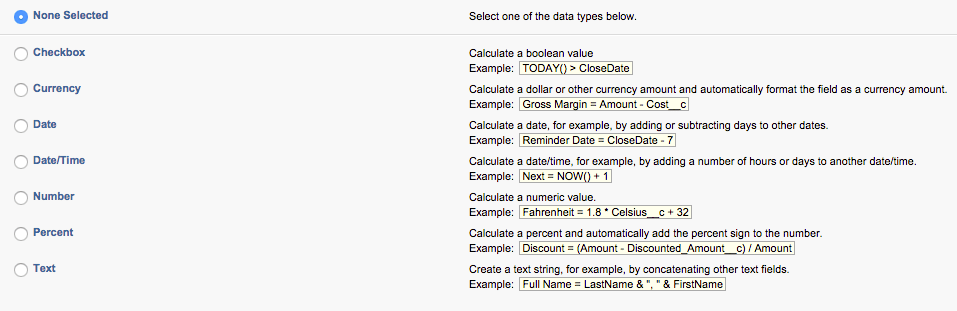# Supercharge Your Experience With Salesforce Formula FieldsSalesforce formula fields can have a huge impact on your Salesforce instance and can provide unrealized, useful information around any object you place them on. They can have a number of applications, such as:

• Perform calculations between multiple number fields
• Display data on a child object from the parent object
• Calculate a certain date between two date fields
• Display different images if a certain criteria is true (like traffic lights)

Formula fields have slightly different characteristics from normal fields, as they are not editable, and their values are set behind the scenes in the object using the formula editor. Formula fields are easy to understand and use logic as opposed to Apex code or any other developer language. If you can handle basic function in an Excel sheet, you can handle formula fields.

Creating a Formula Field

Formula fields are created like any other field by clicking “new” in the field setup of your object and selecting “formula.” Then you are faced with what type of formula field you wish to create.The type of formula field you choose will depend on the kind of output you are allowed to produce. For example, if you choose a number field, you will not be able to introduce date fields into this for a date output. Similarly, if you choose a date field but want to use time values, this will not be allowed.

Layout and Features

After you proceed to the next step, you will be faced with the formula creation page (I would always advise clicking on the Advanced Tab). Take advantage of various features to build simple and complex formulas to display different sets of data on your object. Learning formulas and logic in Salesforce is a good skill to have, as they can be used in various other places like Workflows and Validation Rules.

Insert Field: Insert fields from your object and parent objects. Standard field syntax look like the amount field. Custom fields will always have “__c” appended to the end.

Operators: Operators allows you to test and evaluate data in different ways, such as performing simple mathematical equations or concatenating text.

Functions: Functions allow you to transform data, perform calculations or test data in a more comprehensive way than you can with operators. For example, a common Function is an IF statement; test some data, like “IF Amount > 1000″, display some data if true and some different data if false.Examples include:

Calculate basic commission on Opportunities

Formula: IF (Amount < 10000, Amount * 0.10, Amount * 0.15)

A simple IF statement that looks if the Opportunity Amount is less than 10k, if so the commission is 10%, if it is greater, the commission is 15%. This calculates and displays that commission.

Formula: (IF (AnnualRevenue = 0, 0, 10)) + (IF(ISPICKVAL(Industry, “”) , 0, 10)) + (IF( MobilePhone = “”, 0, 10))

This basic lead score formula looks at whether fields are blank or not and if not, assigns them a score. We can then add these together. Please note the ISPICKVAL function that is necessary if you are using a Picklist field.

Calculate an Accounts Region

Formula: IF(ISBLANK(BillingState), “None”, IF(CONTAINS(“AK:AZ:CA:HA:NV:NM:OR:UT:WA”, BillingState), “West”, IF(CONTAINS(“CO:ID:MT:KS:OK:TX:WY”, BillingState), “Central”, IF(CONTAINS(“CT:ME:MA:NH:NY:PA:RI:VT”, BillingState), “East”, IF(CONTAINS(“AL:AR:DC:DE:FL:GA:KY:LA:MD:MS:NC:NJ:SC:TN:VA:WV”, BillingState), “South”, IF(CONTAINS(“IL:IN:IA:MI:MN:MO:NE:ND:OH:SD:WI”, BillingState), “North”, “Other”))))))

Here’s a great example of a formula field assigning West, Central, East, South, North or Other based on the state. This was taken from the Salesforce Example page.

Using images in formula fields

One of the best things about formula fields is the ability to display images as an output. So in the example above with the Lead Score, instead of outputting a number, we could output an image to display how “hot” the lead is.

Salesforce has a wealth of images that can be used straight out of the box. You can grab a whole list of them here (though you will need to remove some of the URL as instructed). There is also an AppExchange pack you can install to get more.

So carrying on the example with Lead score, here’s a formula with images.